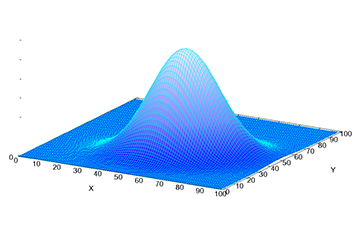# ProbabilityProbability is the study of random phenomenon and the likelihood of random events occuring. An example of a random event includes the outcome of flipping a coin, the uncertain path of a hurricane, or an unexpected epidemic. Probability uses theoretical mathematical tools and has numerous applications in science, engineering and finance.

## Our areas of expertise

Stochastic processes, motion in random media, population genetics, and uncertainty quantification